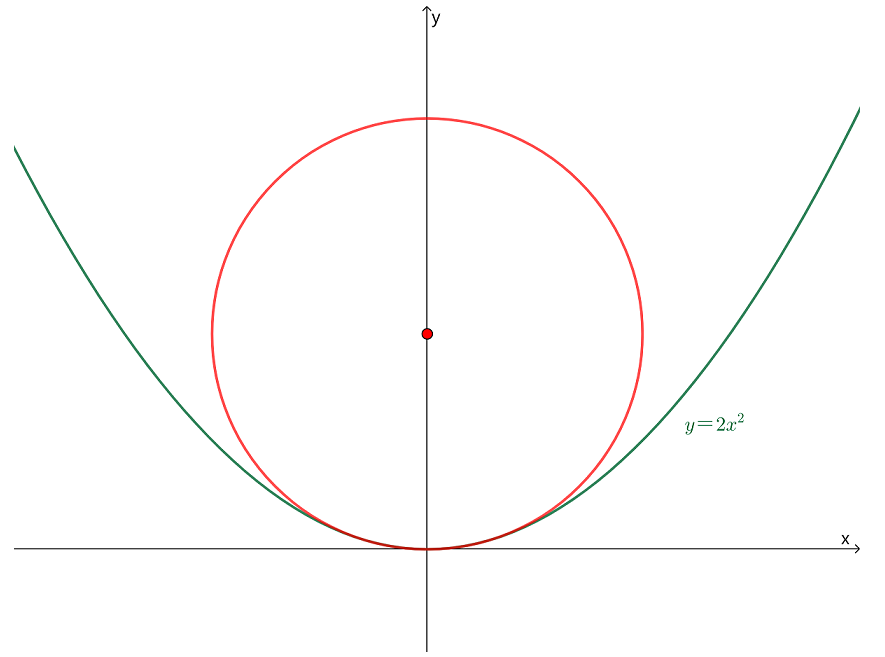安卓手机扫描二维码安装App

﻿$K=$﻿﻿$\dfrac{|y''|}{\sqrt{((1+y'^2)^3}}$﻿

﻿$K=$﻿ ﻿$\dfrac{|2a|}{\sqrt{[1+(2ax+b)^2]^3}}$﻿苹果手机扫描二维码安装App